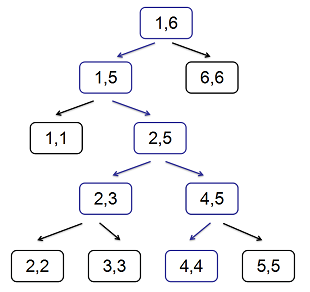# #421. 【集训队作业2018】基础线段树练习题

1.对于所有的 $i\in[l,r]$，将 $A_i$ 变成 $A_i+x$。

2.对于所有的 $i\in[l,r]$，将 $A_i$ 变成 $x$。

3.对于所有的 $i\in[l,r]$，将 $A_i$ 变成 $\lfloor\sqrt{A_i}\rfloor$。

4.对于所有的 $i\in[l,r]$，询问 $A_i$ 的和。$$\begin{equation}\left\{\begin{array}{lr}(0,b_2)&a_2=0;\\(a_1,b_1+b_2)&a_2=1.\\\end{array}\right.\end{equation}$$

1.在节点 $[1,n]$ 将区间 $[l,r]$ 增加 $k$。

2.在节点 $[1,n]$ 将区间 $[l,r]$ 修改为 $k$。

3.在节点 $[1,n]$ 对区间 $[l,r]$ 开根号。

4.询问节点 $[l,r]$ 上的标记。

### 样例一

#### input

0
6 7
1 1 1 1 1 1
5 1 3 2 4
2 2 5 2
4 2 5
1 2 4 1
4 2 5
4 2 3
4 4 4
4 5 5

#### output

0 2
1 0
0 3
0 3
0 2

### 限制与约定

1划分线段树时有 $mid=\lfloor\frac{l+r}{2}\rfloor$
2原线段树树高不超过 $30$
3
4
5
6
7
8
9
10
11
12
13
14
15
16
17
18
19
20

$n = q = 10^5$，$A_i \leq 10^8$